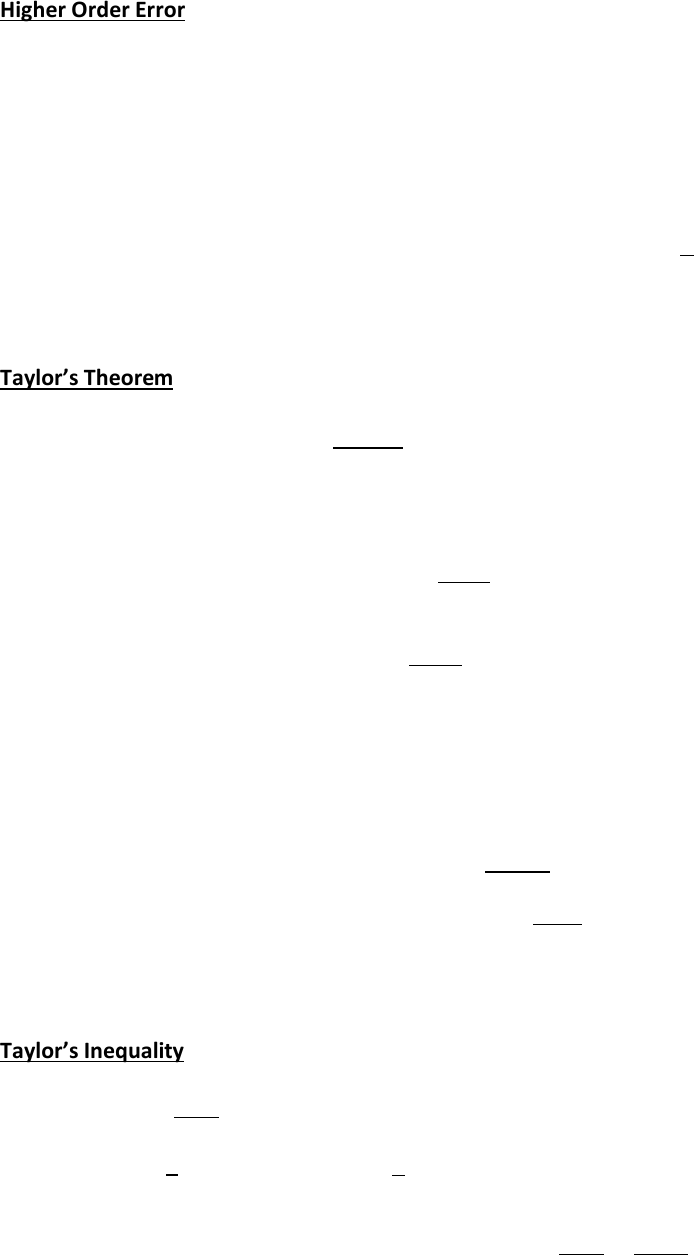# MATH137 Lecture Notes - Lecture 36: Differentiable Function, Inverse Trigonometric Functions

113 views3 pages
School
Department
Course
ProfessorHigher Order Error
Recall for
, we defined the error to be |f(x) -
|. Similarly for the Taylor Polynomial ,
we define the error as |f(x) - |.
The expression f(x) -  is often called the nth degree Taylor Remainder and denoted by 
That is  = f(x) -  so that the error = ||
Again for the linear approximation
, we had the result |f(x) -
|
   provided that
|f ’’| M
For the Taylor polynomial approximation, we have:
Taylor’s Theorem
For an n+1 times differentiable function f on an interval I, if , then there is a c between x and a such
that        
  
Note this is basically a higher other version of the Mean Value Theorem
Recall:        
  
i..e. when n = 0,  = f(a) and we get by Taylor’s theorem:
  
     
      (i.e. MVT)
Furthermore when n =1
        

And Tayler’s theorem says
    
  
   
   
  
Which is our previous result assuming |f ‘’| ≤ M
More generally:
Taylor’s Inequality
Assuming the condition for Taylor’s theorem, if | )| ≤ M, then
Error =  
   
Ex. Approximate using  of f(x) = . Find an upper bound on the error.
Soln:
We previously calculated      

Unlock document

This preview shows page 1 of the document.
Unlock all 3 pages and 3 million more documents.

# Get access

\$10 USD/m
Billed \$120 USD annually
Homework Help
Class Notes
Textbook Notes